• FAQ
• Contact/
/
/
Use the sign test to test the indicated claim. 5) Fourteen
Not my Question
Flag Content

# Question : Use the sign test to test the indicated claim. 5) Fourteen : 1728938

Use the sign test to test the indicated claim.

5) Fourteen people rated two brands of soda on a scale of 1 to 5.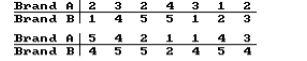At the 5 percent level, test the null hypothesis that the two brands of soda are equally popular.

Use a Kruskal-Wallis test to test the claim that the samples come from populations with equal medians.

6) Listed below are grade averages for randomly selected students with three different categories of high-school background. At the 0.05 level of significance, test the claim that the three groups have the same median grade average.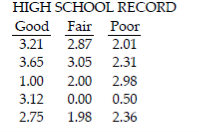Use the Wilcoxon rank-sum test to test the claim that the two independent samples come from populations with equal medians.

7) SAT scores for students selected randomly from two different schools are shown below. Use a significance level of 0.05 to test the claim that the scores for the two schools are from populations with the same median.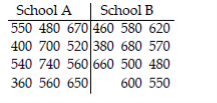Solve the problem.

8) How does the Wilcoxon rank-sum test compare to the corresponding t-test in terms of efficiency, ease of calculations and assumptions required? Are there any kinds of data for which the Wilcoxon rank-sum test can be used but the t-test cannot be used?

The following scatterplot shows the percentage of the vote a candidate received in the 2004 senatorial elections according to the voter’s income level based on an exit poll of voters conducted by CNN. The income levels 1 -8 correspond to the following income classes: 1=Under \$15,000; 2=\$15-30,000; 3=\$30-50,000; 4=\$50-75,000; 5=\$75-100,000 ; 6=\$100-150,000; 7=\$150-200,000; 8=\$200,000 or more.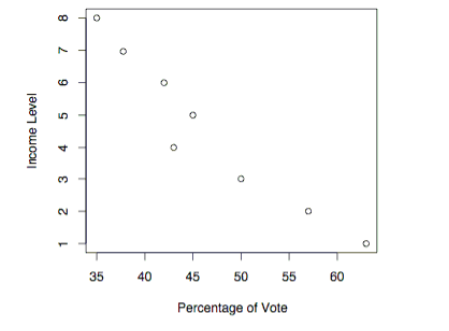9) Use the election scatterplot to the find the critical values corresponding to a 0.01 significance level used to test the null hypothesis of ρs = 0.

A) -0.881 and 0.881 B) -0.881

C) -0.738 and 0.738 D) 0.881

Use the runs test to determine whether the given sequence is random. Use a significance level of 0.05.

10) The sequence of numbers below represents the maximum temperature (in degrees

Fahrenheit) in July in one U.S. town for 30 consecutive years. Test the sequence for randomness above and below the median.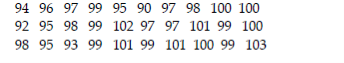Find the critical value. Assume that the test is two-tailed and that n denotes the number of pairs of data.

11) n =20, α = 0.01

A) -0.570 B) 0.570 C) ±0.447 D) ±0.570

## Solution 5 (1 Ratings )

Solved
Statistics 1 Year Ago 21 Views